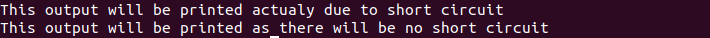# Short-Circuiting in C++ and Linux

Short-circuiting is one of the optimization steps of the compiler, in this step unnecessary calculation is avoided during the evaluation of an expression. Expression is evaluated from left to right. It works under certain cases when the value of the expression can be calculated certainly by only evaluating parts of the expression.

Short-circuiting in C++
In C++ short-circuiting occurs while evaluating ‘&&’ (AND) and ‘||'(OR) logical operators. While evaluating ‘&&’ operator if the left-hand side of ‘&&’ gives false, then the expression will always yield false irrespective of the value of the right-hand side of ‘&&’, so checking right-hand side of ‘&&’ makes no sense. So, in this situation evaluation of the right-hand side is avoided by the compiler. Similarly, in the case of logical OR ‘||’ operation when the left-hand side gives ‘true’, the result of the expression will always be true irrespective of the value of the right-hand side.

Short-circuiting using AND(&&) and OR(||) logical operator.

## C++

 `#include ` `using` `namespace` `std;`   `int` `main()` `{` `    ``// Short circuiting` `    ``// logical "||"(OR)` `    ``int` `a = 1, b = 1, c = 1;`   `    ``// a and b are equal` `    ``if` `(a == b || c++) {` `        ``cout << ``"Value of 'c' will"` `             ``<< ``"  not increment due"` `             ``<< ``" to short-circuit"` `             ``<< endl;` `    ``}` `    ``else` `{` `        ``cout << ``"Value of 'c' "` `             ``<< ``" is incremented as there"` `             ``<< ``" is no short-circuit"` `             ``<< endl;` `    ``}`   `    ``// Short circuiting` `    ``// logical "&&"(OR)`   `    ``if` `(a == b && c++) {` `        ``cout << ``"Value of 'c' will"` `             ``<< ``" increment as there"` `             ``<< ``" is no short circuit"` `             ``<< endl;` `    ``}` `    ``else` `{` `        ``cout << ``"Value of 'c' will"` `             ``<< ``" not increment due to short circuit"` `             ``<< endl;` `    ``}` `}`

Output:

```Value of 'c' will  not increment due to short-circuit
Value of 'c' will increment as there is no short circuit```

Short Circuiting in Linux
In Linux, short-circuiting takes place while evaluating ‘&&’ and ‘||’ operators just like C++.

Logical AND(&&) short circuiting:

## PHP

 `if` `[[ ``"\$1"` `-gt 5 ]] && [[ ``"\$1"` `-lt 10 ]]; then` `    ``echo` `"This output will not be printed"` `else` `    ``echo` `"This output will be printed"` `         ``" actually due to short circuit"`     `fi`     `if` `[[ ``"\$1"` `-lt 5 ]] && [[ ``"\$1"` `-lt 10 ]]; then` `    ``echo` `"This output will be printed"` `         ``" as there will be no short circuit"` `else` `    ``echo` `"This output will be printed"` `         ``" actually due to short circuit"`     `fi`

Output:Logical OR(||) short-circuiting:

## PHP

 `if` `[[ ``"\$1"` `-lt 5 ]] || [[ ``"\$1"` `-gt 10 ]]; then` `    ``echo` `"This output will be printed"` `         ``" actually due to short circuit"`  `else` `    ``echo` `"This output will be printed"` `         ``" as there will be no short circuit"`   `fi`   `if` `[[ ``"\$1"` `-gt 5 ]] || [[ ``"\$1"` `-lt 10 ]]; then` `    ``echo` `"This output will be printed"` `         ``" as there will be no short circuit"` `else` `    ``echo` `"This output will be printed"` `         ``" actually due to short circuit"`     `fi`

Output:Note: ‘&&’ operator is often used as a replacement to ‘if’ statement.

For example, to check for the existence of a file you could use either of the below:

• if [[ -e “\$filename” ]]; then
echo “exists”
fi
• [[ -e “\$filename” ]] && echo “exists”

In this case, bash first executes the expression on the left of the &&(and). If that expression has a non-zero return value (i.e. it failed), then there is no need to evaluate the right side of the && because the overall exit status is already known to be non-zero since both sides have to return a success indicator for a logical and to return success.

Whether you're preparing for your first job interview or aiming to upskill in this ever-evolving tech landscape, GeeksforGeeks Courses are your key to success. We provide top-quality content at affordable prices, all geared towards accelerating your growth in a time-bound manner. Join the millions we've already empowered, and we're here to do the same for you. Don't miss out - check it out now!

Previous
Next